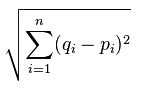# Machine Learning – Tutorial 15

## Euclidean Distance

https://pythonprogramming.net/euclidean-distance-machine-learning-tutorial/This stuff makes my head hurt….just look at this formula….WTF man!

Basically, it’s just the square root of the sum of the distance of the points from each other, squared….easy

So in Python this translates into:-

```plot1 = [1,3]
plot2 = [2,5]
euclidean_distance = sqrt( (plot1-plot2)**2 + (plot1-plot2)**2 )
```